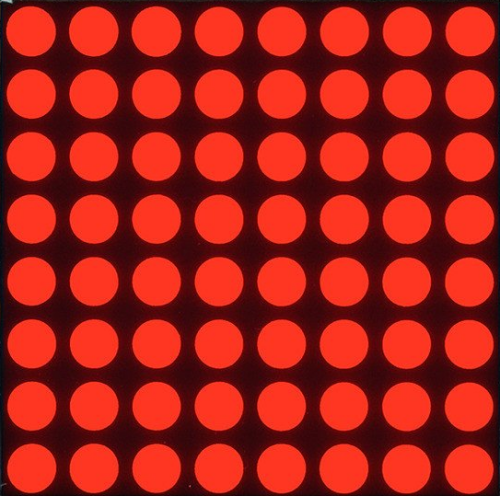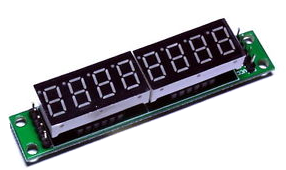Creative Science Centre

## MAX7129_Driver

This device can drive a single 8 digit (digital tube) 7 segment display or one LED Matrix 8x8. The shift register has an output so more then one device can be used.or// #include "http://www.byvac.com/mBlib/flb/Library/2016/lib_max7219.bas"

The library does require a single dimension array. The size should be tiles*8

• max72.init(*cs(),tiles) A tile is either one 8x8 dot matrix or one 8 digit display, CS is the chip select pin specified as a contant, for example if RA2 was used then constant {PORTA(PORT),2}
• max72.cls() Switches off all leds on all tiles
• max72.store_init(*store(),len) Initialise the array (called store) size is the size of the array
• max72.loadStore(*store()) writes the whole store to the MAX7192 chain

## Dot Matrix Driver - with font

Uses the MAX7192 driver so this also requires a singe dimension array.

// #include "http://www.byvac.com/mBlib/flb/Library/2016/lib_matrix.bas"

• matrix.init(*store(),*cs(),tiles) Store is a single dimension array, cs() is the chip select line as described in the MAX7219 library above and tiles are the number of 8x8 matrix units.
• matrix.colSetByteH(*store(),column,data) Will place a byte (data) in the given column which will illuminate the column. The column begins at 0 and ends at (tiles * 8) - 1. The MSB is at the top of the tile.
• matrix.colSetByteL(*store(),column,data) as above but the MSB is at the bottom of the tile.
• matrix.colGetByteH(*store(),column) Gets the byte of data at the column stored by matrix.colSetByteH(*store(),column,data)
• matrix.pix(*store(),row, col, onn) Turns on (onn=1) or off (onn=0) one pixel (LED) at the specified row and column. Row 0 is at the top and row 7 is at the bottom. The column begins at 0 and ends at (tiles * 8) - 1. The MSB is at the top of the tile.
• matrix.putc(*store(),col,c) Puts a character (c) at the given column and returns the next column after the character.
• matrix.puts(*store(),col,s\$) Writes a string starting at the given column.